How to use COLUMN function in Microsoft Excel

COLUMN function returns the number of the current column where the function is located or the column number of a given cell reference.

COLUMN function has only one argument which is “reference”. It is a reference to a cell or range of cells for which you want to return the column number. If the reference is omitted, function assumes that the reference is the cell address in which the COLUMN function has been entered.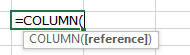Let’s take examples and understand the applications of COLUMN function.

We have data in which we have taken 2 different types of examples; let’s have a look at the first example:-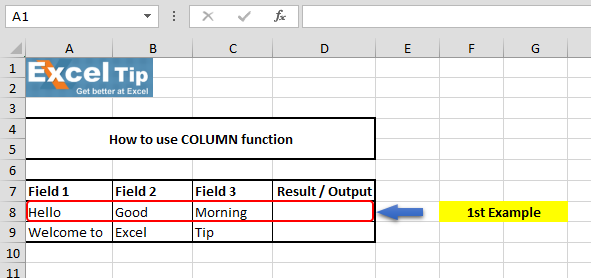In our first example, we will omit the reference argument and will tell the function to return the column number based on the function’s location in the excel sheet.

• Enter the function in cell D8
• =COLUMN(), Press Enter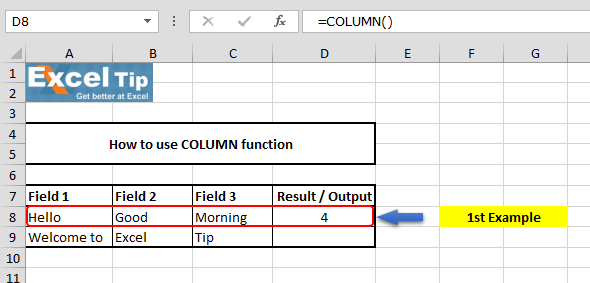It returns 4 in cell D8 since the function is located in column D which is the fourth column of the worksheet.

2nd Example:-

In this example, we will return column number on the basis of the entered cell reference.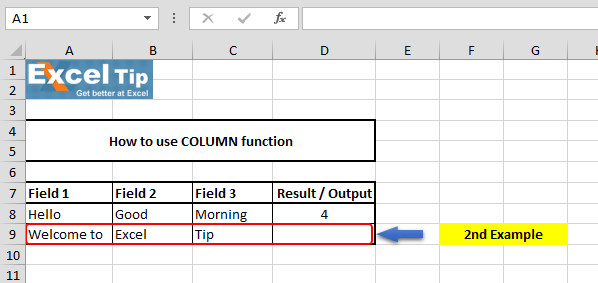• Enter the function in cell D9
• =COLUMN(F2), Press Enter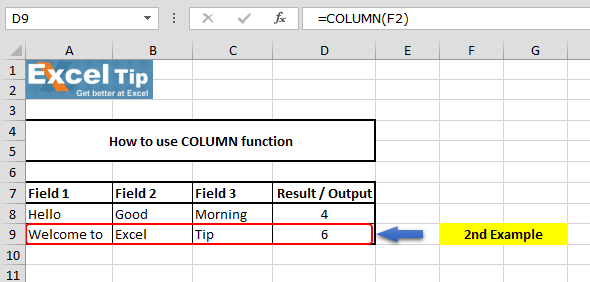We get 6 as the column number since the cell F2 is in the sixth column of the worksheet. By changing the cell reference, column number will get changed accordingly.

So, this is how COLUMN function works in Microsoft Excel.Video: How to use COLUMN function in Microsoft Excel

Click on the video link for quick reference to the use of COLUMN function. Subscribe to our new channel and keep learning with us!